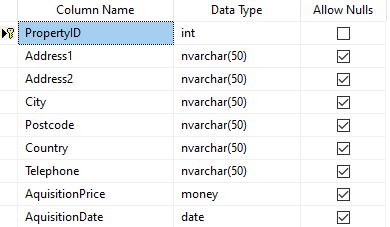# Sums - How to calculate 'year to date' or running totals

There can often be the requirement to calculate running totals and to display the results in a gallery or data table control. This post describes the formula to carry out this task.

The ability to calculate running totals can be particularly important for reporting and financial applications, especially when we want to display 'year to date' figures. In this post, we'll walk through one way to carry out this task.

## Walkthrough - How to calculate running totals

As an example, we'll take the example of a property table. There are 2 prominent fields - 'acquisition date' and 'acquisition price'.A typical use case scenario is to display records where the 'acquisition date' matches the current year, and to show a running total of the acquisition price.

We can accomplish this by adding a gallery control to a screen and setting the items property to the formula beneath:

`With({data: Property},     ForAll(Sequence(CountRows(data)),            Patch(Last(FirstN(data,Value)),                  {                      RowNumber:Value,                      RunningTotal:Sum(FirstN(data,Value),AquisitionPrice)                  }            )    ))`

This technique applies an adaption of a technique to generate sequences row numbers. But instead of generating a row number, we calculate a running sum. There are more details in the link beneath:

To apply this technqiue, we would replace the 'data' variable inside the 'With' block with the target data source. We could replace this with a call to the Filter function to return rows that match specific criteria (for example, records with a date that match the current year).

The formula applies the ForAll function against a sequence with a length that matches the row count of source data. This formula effectively applies a sequential number against each record in the data source. For each record in the data source, we patch an additional field called 'RunningTotal'. We set the value of this field to the output of a call to the Sum function. The Sum function here sums 'aquisition price' of all the records up to the current row.

The screenshot beneath illustrates the appearance of this technique.## Are there any caveats to this technique?

The caveat to this technique is that Power Apps applies the 'running total' against the maximum number of records that it can fetch in one go, which corresponds to the number of records that are defined in the 'data row limit' setting of the app. The maximum value that we can apply here is 2,000 records. Therefore, if we want to calculate a running total against more than 2,000 records, we must collect all the records into a collection, and apply the technique against the collection,.

## Conclusion

The ability to calculate running totals can be particularly important for reporting and financial applications, especially when we want to display 'year to date' figures. This post described a formula to accomplish this task.
•   Categories:
• sum
Related posts